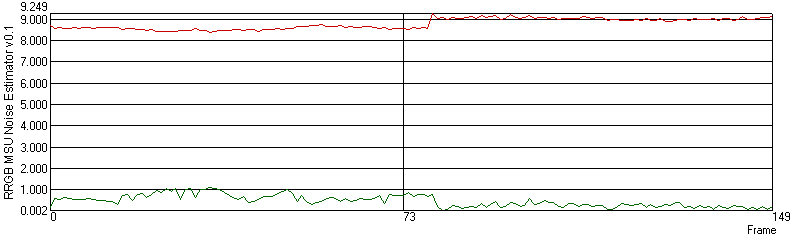# MSU Noise Estimation Metric (NE)

• Project, idea: Dr. Dmitriy Vatolin, Sergey Grishin
• Algorithm, implementation: Kumok Boris
• Updating and additions: Sheludko Victor, Sergey Putilin, Sergey Grishin

## Common idea

Metrics Noise Estimator is intended for calculation of noise level for each frame of video sequence.

## Changelog

[!] — Known bug
[+] — New Feature
[*] — Other

0.3
[*] Incorrect processing of non-standard resolution video bug fixed

0.2
[*] Bug with incorrect (identical) values for some videos is fixed.
[*] Command line name is changed (became easier to use)

0.1
[*] First released version.

## Usage

The metrics realizes three various algorithms of definition of noise level:

• Block-Based

The choice of which algorithm to use can be made in Settings. To algorithms there correspond figures from 0 up to 2.

## Visualization

Visualization of the metrics does not carry any information.

## Plots

By results of job of the metrics the plot of frame-accurate value of noise level is constructed. Final value of the metrics is average arithmetic of all frame-accurate values.Plot's example

## Algorithm

For each frame do HAAR wavelet decomposition. Than evaluate median of HH-component’s absolute values. Final value of the metrics is the normalized median.

### Block-Based

Frames are tessellated into a number of 8x8 blocks. Standard deviations of intensity (measures of intensity variation) are computed for all the blocks and sorted. The block with the smallest standard deviation has the least change of intensity. The smaller the standard deviation, the smother the block. The intensity variation of a smooth block may be due to noise, in which the standard deviation of the block is close to that of the Gaussian noise added. Normalized average arithmetic values of 30 % of all blocks with the least values grows is the final value of the metric.